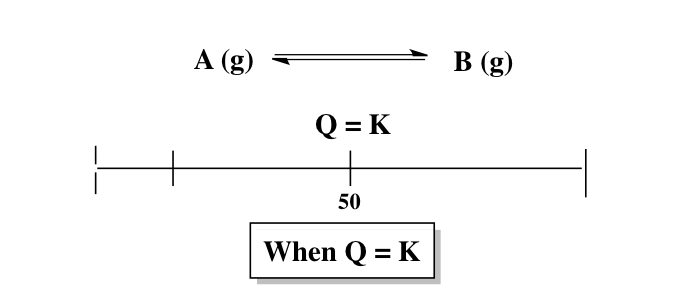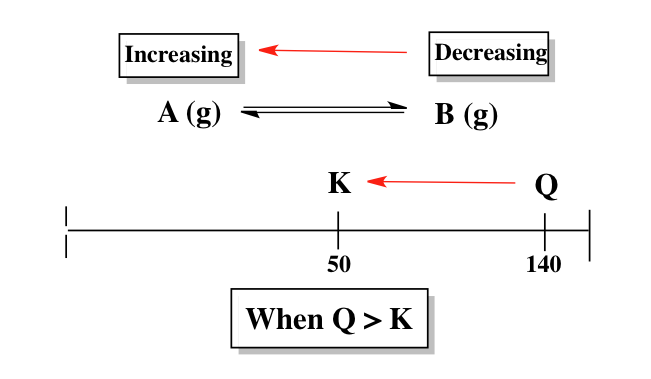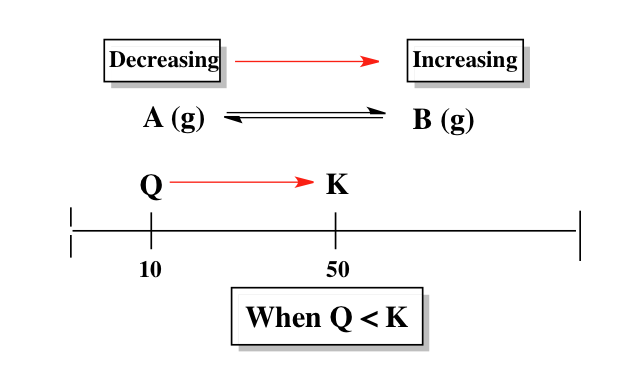Start typing, then use the up and down aroows to select an option from the list.

# Analytical Chemistry

Learn the toughest concepts covered in your Analytical Chemistry class with step-by-step video tutorials and practice problems.

Chemical Equilibrium

The reaction quotient, Q, is useful in determining if a chemical reaction is at equilibrium.

## The Reaction Quotient

1

### concept

The Reaction Quotient4m
Play a video:
0

When Q = K then our chemical reaction is at equilibrium and no shifting will occur.If Q is larger than K then the reaction will shift in the reverse direction to attain equilibrium.If Q is smaller than K then the reaction will shift in the forward direction to attain equilibrium.2

### example

The Reaction Quotient3m
Play a video:
0

## Reaction Quotient Calculations

3

### example

Reaction Quotient Calculations 12m
Play a video: